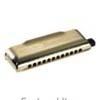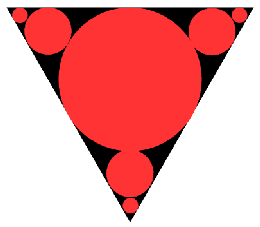#### You may also like### Generally Geometric

Generalise the sum of a GP by using derivatives to make the coefficients into powers of the natural numbers.### Harmonically

Is it true that a large integer m can be taken such that: 1 + 1/2 + 1/3 + ... +1/m > 100 ?

# Circles Ad Infinitum

##### Age 16 to 18 Challenge Level:

A circle of radius 1 cm is inscribed in an equilateral triangle. A smaller circle is inscribed at each vertex touching the first circle and tangent to the two 'containing' sides of the triangle. This process is continued ad infinitum....What is the sum of the circumferences of all the circles?

What is the sum of their areas?

Adding all the circumferences or adding all the areas, which sum grows faster?